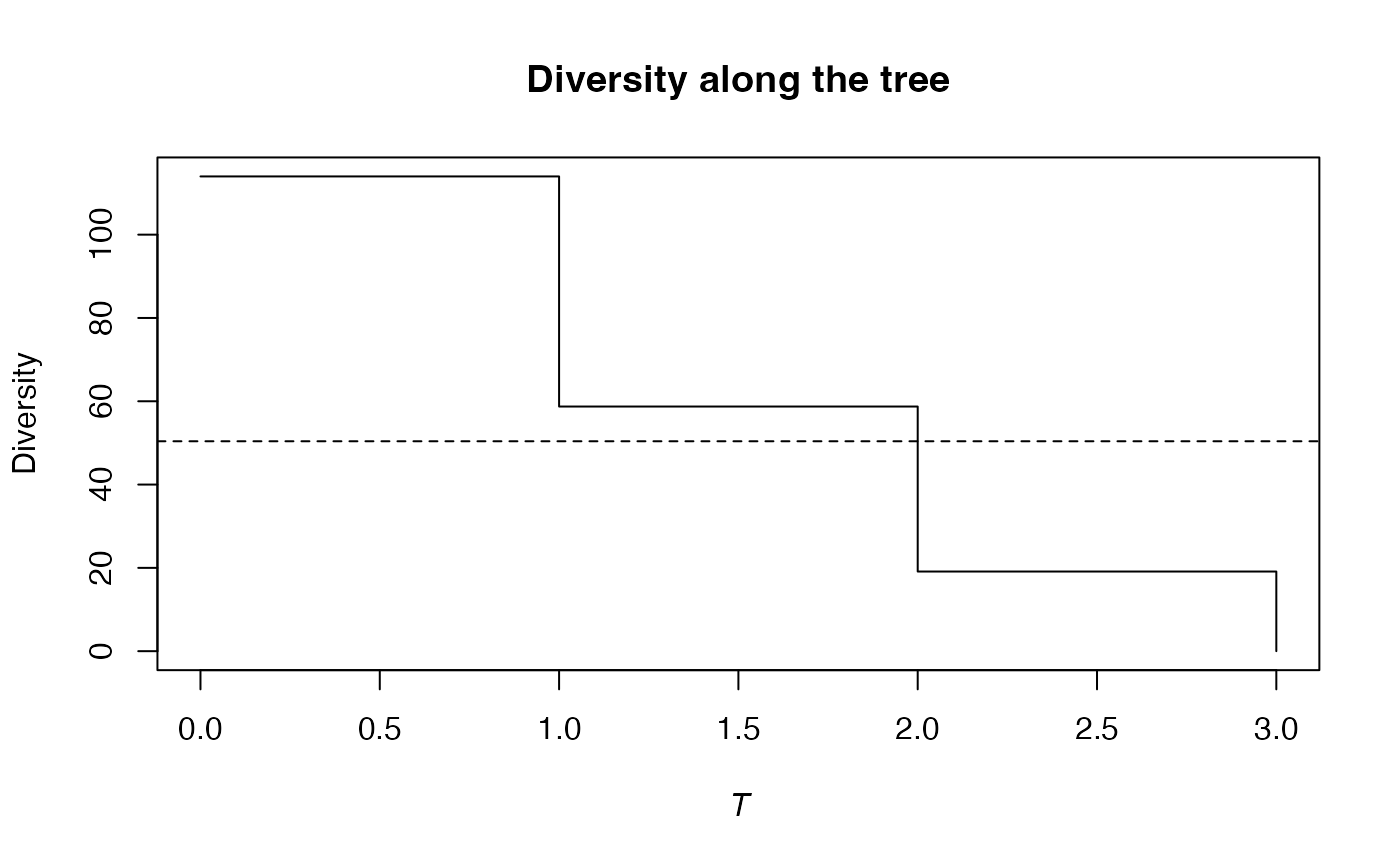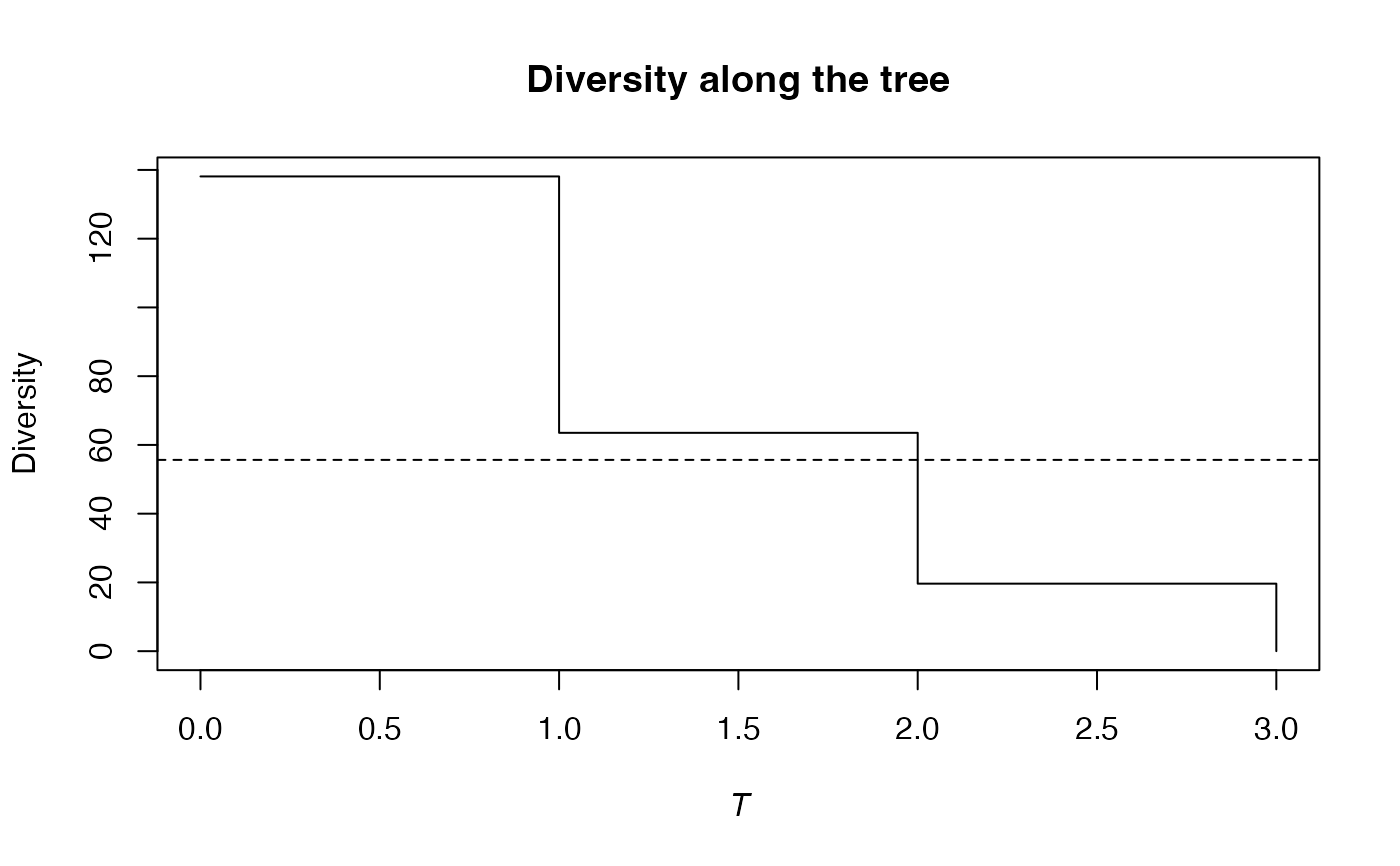Calculates the phylogenetic diversity of order $$q$$ of a probability vector.

PhyloDiversity(NorP, q = 1, Tree, Normalize = TRUE, ...)
bcPhyloDiversity(Ns, q = 1, Tree, Normalize = TRUE, Correction = "Best",
CheckArguments = TRUE)
# S3 method for ProbaVector
PhyloDiversity(NorP, q = 1, Tree, Normalize = TRUE,
..., CheckArguments = TRUE, Ps = NULL)
# S3 method for AbdVector
PhyloDiversity(NorP, q = 1, Tree, Normalize = TRUE,
Correction = "Best", ..., CheckArguments = TRUE, Ns = NULL)
# S3 method for integer
PhyloDiversity(NorP, q = 1, Tree, Normalize = TRUE,
Correction = "Best", ..., CheckArguments = TRUE, Ns = NULL)
# S3 method for numeric
PhyloDiversity(NorP, q = 1, Tree, Normalize = TRUE,
Correction = "Best", ..., CheckArguments = TRUE, Ps = NULL, Ns = NULL)
is.PhyloDiversity(x)
# S3 method for PhyloDiversity
summary(object, ...)

## Arguments

Ps

A probability vector, summing to 1.

Ns

A numeric vector containing species abundances.

NorP

A numeric vector, an integer vector, an abundance vector (AbdVector) or a probability vector (ProbaVector). Contains either abundances or probabilities.

q

A number: the order of diversity. Default is 1.

Tree

An object of class hclust, phylo, phylog or PPtree. The tree must be ultrametric.

Normalize

If TRUE (default), the Total diversity is not affected by the height of the tree.
If FALSE, it is proportional to the height of the tree.

Correction

A string containing one of the possible corrections: "None" (no correction), "ChaoShen", "Grassberger", "Holste", "Bonachela" or "Best", the default value.

CheckArguments

Logical; if TRUE, the function arguments are verified. Should be set to FALSE to save time when the arguments have been checked elsewhere.

x

An object to be tested or plotted

object

A PhyloDiversity object to be summarized.

...

Additional arguments to be passed to the generic methods.

## Details

The phylogenetic entropy is its generalization of HCDT entropy to unequal species distances (Pavoine et al., 2009).

Diversity is obtained by transforming generalized entropy.

Bias correction requires the number of individuals to estimate sample Coverage. Use bcPhyloDiversity and choose the Correction.

The functions are designed to be used as simply as possible. PhyloDiversity is a generic method. If its first argument is an abundance vector, an integer vector or a numeric vector which does not sum to 1, the bias corrected function bcPhyloDiversity is called. Explicit calls to bcPhyloDiversity (with bias correction) or to PhyloDiversity.ProbaVector (without correction) are possible to avoid ambiguity. The .integer and .numeric methods accept Ps or Ns arguments instead of NorP for backward compatibility.

## Note

The tree must contain all species of the probability vector. If it contains extra species, computation time will just be increased.

## Value

An object of class PhyloDiversity is a list:

Distribution

The distribution used to calculate diversity

Function

The function used to calculate diversity

Tree

The functional or phylogenetic tree used to calculate diversity

Normalized

Logical. Indicates whether phylodiversity is normalized or proportional to the height of the tree.

Type

The type of diversity ("alpha", "beta" or "gamma").

Order

The order of diversity $$q$$.

Cuts

A named vector containing values of neutral diversity along the tree. Names are cut ends, i.e. the ends of intervals (the first interval starts at 0 for leaves, the max value is the height of the tree).

Total

A value equal the total diversity (obtained by transforming the total normalized entropy), multiplied by the tree height if Normalize is FALSE.

is.PhyloDiversity returns TRUE if the object is of class PhyloDiversity. summary.PhyloDiversity returns a summary of the object's value. PhyloDiversity objects can be plotted by plot.PhyloValue because PhyloDiversity objects are also of class PhyloValue.

PhyloEntropy, Diversity

## Examples

# Load Paracou data (number of trees per species in two 1-ha plot of a tropical forest
#      and their taxonomy)
data(Paracou618)
# Ps is the vector of probabilities
Ps <- as.ProbaVector(Paracou618.MC$Ps) # Calculate the phylogenetic Shannon diversity of the plot summary(PhyloDiversity(Ps, 1, Paracou618.Taxonomy) -> d) #> alpha or gamma phylogenetic or functional diversity of order 1 of distribution #> - #> with correction: None #> Phylogenetic or functional diversity was calculated according to the tree #> Paracou618.Taxonomy #> #> Diversity is normalized #> #> Diversity equals: 50.39747 plot(d)# Ns is the vector of abundances of the metacommunity Ns <- as.AbdVector(Paracou618.MC$Ns)
# Calculate the phylogenetic Shannon diversity of the plot
summary(bcPhyloDiversity(Ns, 1, Paracou618.Taxonomy, Correction = "Best") -> d)
#> alpha or gamma phylogenetic or functional diversity of order 1 of distribution
#> -
#>  with correction: Best
#> Phylogenetic or functional diversity was calculated according to the tree
#> Paracou618.Taxonomy
#>
#> Diversity is normalized
#>
#> Diversity equals: 55.64502
plot(d)